Open in App
Not now

# How to build a basic CRUD app with Node.js and ReactJS ?

• Difficulty Level : Hard
• Last Updated : 10 Apr, 2022

In this article, we will create a basic Student app from scratch.

App functionality:

• Create a new student
• Update an existing student
• Show students list
• Delete a student

REST API in this project:

First of all, we will work on the frontend part of our application using React.js.

Create React Application and installing modules

Step 1: Let’s start building the Front-end part with React. To create a new React App, enter the following code into terminal and hit enter.

`npx create-react-app mern-stack-crud`

Step 2: Move into the React project folder.

`cd mern-stack-crud`

Step 3: To run the React App, run the following command:

`npm start`

This command opens the React App to the browser on the following URL: http://localhost:3000/

Step 4: To build the React App we need to install some external modules.

To install, run the following code on the terminal.

npm i react-bootstrap@next bootstrap@5.1.0 react-router-dom axios formik yup

Step 5: Creating Simple React Components – In this step we will create some React Components to manage student data.

Head over to src folder, make a folder and name it Components and within that directory create the following components.

• StudentForm.js – Reusable Student form
• create-student.component.js – Responsible for create new student
• edit-student.component.js – Responsible for update student data
• student-list.component.js – Responsible for display all student
• StudentTableRow.js  – Responsible for display a single student

Project Structure: It will look like the following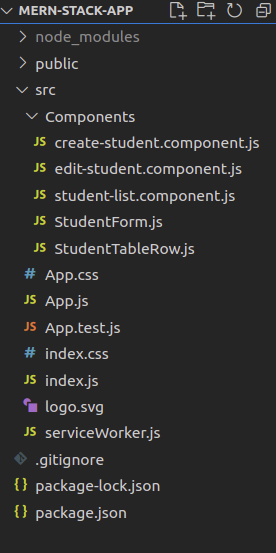front-end project structure

Step 6: Create student form – In this step, we will build a reusable student form with Formik and React-Bootstrap. This form has all the necessary fields to enter student details. We have also made client-side form validation with Yup. In the future, we will use this component for creating and update a student. Go to src/Components/StudentForm.js and write the following code.

## StudentForm.js

 `import React from ``"react"``; ` `import * as Yup from ``"yup"``; ` `import { Formik, Form, Field, ErrorMessage } from ``"formik"``; ` `import { FormGroup, FormControl, Button } from ``"react-bootstrap"``; ` ` `  `const StudentForm = (props) => { ` `  ``const validationSchema = Yup.object().shape({ ` `    ``name: Yup.string().required(``"Required"``), ` `    ``email: Yup.string() ` `      ``.email(``"You have enter an invalid email address"``) ` `      ``.required(``"Required"``), ` `    ``rollno: Yup.number() ` `      ``.positive(``"Invalid roll number"``) ` `      ``.integer(``"Invalid roll number"``) ` `      ``.required(``"Required"``), ` `  ``}); ` `  ``console.log(props); ` `  ``return` `( ` `    ``
` `      `` ` `        ``
` `          `` ` `            `` ` `            `` ` `          `` ` `          `` ` `            `` ` `            `` ` `          `` ` `          `` ` `            `` ` `            `` ` `          `` ` `          `` ` `        `` ` `      ``
` `    ``
` `  ``); ` `}; ` ` `  `export ``default` `StudentForm; `

Step 7: Create a new student: In this step, we will create a component to add a new student.  We have already created a StudentForm component to enter student details. Now, it’s time to use this component. Go to src/Components/create-student.component.js and write the following code.

## create-student.component.js

 `// CreateStudent Component for add new student ` ` `  `// Import Modules ` `import React, { useState, useEffect } from ``"react"``; ` `import axios from ``'axios'``; ` `import StudentForm from ``"./StudentForm"``; ` ` `  `// CreateStudent Component ` `const CreateStudent = () => { ` `  ``const [formValues, setFormValues] =  ` `    ``useState({ name: ``''``, email: ``''``, rollno: ``''` `}) ` `  ``// onSubmit handler ` `  ``const onSubmit = studentObject => { ` `    ``axios.post( ` `'http://localhost:4000/students/create-student'``,  ` `    ``studentObject) ` `      ``.then(res => { ` `        ``if` `(res.status === 200) ` `          ``alert(``'Student successfully created'``) ` `        ``else` `          ``Promise.reject() ` `      ``}) ` `      ``.``catch``(err => alert(``'Something went wrong'``)) ` `  ``} ` `   `  `  ``// Return student form ` `  ``return``( ` `    `` ` `      ``Create Student ` `    `` ` `  ``) ` `} ` ` `  `// Export CreateStudent Component ` `export ``default` `CreateStudent`

Step 8: Update student’s details: In this section, we will create a component to update details. We have reusable StudentForm component, let’s use it again. We will fetch student details to reinitialise form. Go to src/Components/edit-student.component.js and write the following code.

## edit-student.component.js

 `// EditStudent Component for update student data ` ` `  `// Import Modules ` `import React, { useState, useEffect } from ``"react"``; ` `import axios from ``"axios"``; ` `import StudentForm from ``"./StudentForm"``; ` ` `  `// EditStudent Component ` `const EditStudent = (props) => { ` `  ``const [formValues, setFormValues] = useState({ ` `    ``name: ``""``, ` `    ``email: ``""``, ` `    ``rollno: ``""``, ` `  ``}); ` `   `  `  ``//onSubmit handler ` `  ``const onSubmit = (studentObject) => { ` `    ``axios ` `      ``.put( ` `        ``"http://localhost:4000/students/update-student/"` `+ ` `          ``props.match.params.id, ` `        ``studentObject ` `      ``) ` `      ``.then((res) => { ` `        ``if` `(res.status === 200) { ` `          ``alert(``"Student successfully updated"``); ` `          ``props.history.push(``"/student-list"``); ` `        ``} ``else` `Promise.reject(); ` `      ``}) ` `      ``.``catch``((err) => alert(``"Something went wrong"``)); ` `  ``}; ` ` `  `  ``// Load data from server and reinitialize student form ` `  ``useEffect(() => { ` `    ``axios ` `      ``.get( ` `        ``"http://localhost:4000/students/update-student/"`  `        ``+ props.match.params.id ` `      ``) ` `      ``.then((res) => { ` `        ``const { name, email, rollno } = res.data; ` `        ``setFormValues({ name, email, rollno }); ` `      ``}) ` `      ``.``catch``((err) => console.log(err)); ` `  ``}, []); ` ` `  `  ``// Return student form ` `  ``return` `( ` `    `` ` `      ``Update Student ` `    `` ` `  ``); ` `}; ` ` `  `// Export EditStudent Component ` `export ``default` `EditStudent;`

Step 9: Display list of students: In this step, we will build a component to display the student details in a table.  We will fetch student’s data and iterate over it to create table row for every student. Go to src/Components/student-list.component.js and write the following code.

## student-list.component.js

 `import React, { useState, useEffect } from ``"react"``; ` `import axios from ``"axios"``; ` `import { Table } from ``"react-bootstrap"``; ` `import StudentTableRow from ``"./StudentTableRow"``; ` ` `  `const StudentList = () => { ` `  ``const [students, setStudents] = useState([]); ` ` `  `  ``useEffect(() => { ` `    ``axios ` `      ``.get(``"http://localhost:4000/students/"``) ` `      ``.then(({ data }) => { ` `        ``setStudents(data); ` `      ``}) ` `      ``.``catch``((error) => { ` `        ``console.log(error); ` `      ``}); ` `  ``}, []); ` ` `  `  ``const DataTable = () => { ` `    ``return` `students.map((res, i) => { ` `      ``return` `; ` `    ``}); ` `  ``}; ` ` `  `  ``return` `( ` `    ``
` `      `` ` `        ``
NameEmailRoll NoAction
` `          `` ` `            `` ` `            `` ` `            `` ` `            `` ` `          `` ` `        `` ` `        ``{DataTable()} ` `      `` ` `    `` ` `  ``); ` `}; ` ` `  `export ``default` `StudentList;`

Step 10: Display a single student: In this step, we will return table row which is responsible to display student data. Go to src/Components/StudentTableRow.js and write the following code.

## StudentTableRow.js

 `import React from ``"react"``; ` `import { Button } from ``"react-bootstrap"``; ` `import { Link } from ``"react-router-dom"``; ` `import axios from ``"axios"``; ` ` `  `const StudentTableRow = (props) => { ` `  ``const { _id, name, email, rollno } = props.obj; ` ` `  `  ``const deleteStudent = () => { ` `    ``axios ` `      ``.``delete``( ` `"http://localhost:4000/students/delete-student/"` `+ _id) ` `      ``.then((res) => { ` `        ``if` `(res.status === 200) { ` `          ``alert(``"Student successfully deleted"``); ` `          ``window.location.reload(); ` `        ``} ``else` `Promise.reject(); ` `      ``}) ` `      ``.``catch``((err) => alert(``"Something went wrong"``)); ` `  ``}; ` ` `  `  ``return` `( ` `    ``
{name}{email}{rollno} ` `        `` ` `          ``Edit ` `        `` ` `        `` ` `      ``
` `      `` ` `      `` ` `      `` ` `      `` ` `    `` ` `  ``); ` `}; ` ` `  `export ``default` `StudentTableRow;`

Step 11: Edit App.js: Finally, include the menu to make routing in our MERN Stack CRUD app. Go to src/App.js and write the following code.

## App.js

 `// Import React ` `import React from ``"react"``; ` ` `  `// Import Bootstrap ` `import { Nav, Navbar, Container, Row, Col }  ` `        ``from ``"react-bootstrap"``; ` `import ``"bootstrap/dist/css/bootstrap.css"``; ` ` `  `// Import Custom CSS ` `import ``"./App.css"``; ` ` `  `// Import from react-router-dom ` `import { BrowserRouter as Router, Switch, ` `    ``Route, Link } from ``"react-router-dom"``; ` ` `  `// Import other React Component ` `import CreateStudent from  ` `    ``"./Components/create-student.component"``; ` `import EditStudent from  ` `    ``"./Components/edit-student.component"``; ` `import StudentList from  ` `    ``"./Components/student-list.component"``; ` ` `  `// App Component ` `const App = () => { ` `  ``return` `( ` `    `` ` `      ``
` `        ``
` `          `` ` `            `` ` `              `` ` `                `` ` `                  ``React MERN Stack App ` `                `` ` `              `` ` ` `  `              `` ` `            `` ` `          `` ` `        ``
` ` `  `        `` ` `          `` ` `            ``
` `              ``
` `                `` ` `                  `` ` `                  `` ` `                  `` ` `                  `` ` `                `` ` `              ``
` `            `` ` `          `` ` `        `` ` `      `` ` `    `` ` `  ``); ` `}; ` ` `  `export ``default` `App;`

Step 12: Add style – Go to src/App.css and write the following code.

## App.css

 `.wrapper { ` `  ``padding-top``: ``30px``; ` `} ` ` `  `body h``3` `{ ` `  ``margin-bottom``: ``25px``; ` `} ` ` `  `.navbar-brand a { ` `  ``color``: ``#ffffff``; ` `} ` ` `  `.form-wrapper, ` `.table-wrapper { ` `  ``max-width``: ``500px``; ` `  ``margin``: ``0` `auto``; ` `} ` ` `  `.table-wrapper { ` `  ``max-width``: ``700px``; ` `} ` ` `  `.edit-link { ` `  ``padding``: ``7px` `10px``; ` `  ``font-size``: ``0.875``rem; ` `  ``line-height``: ``normal``; ` `  ``border-radius: ``0.2``rem; ` `  ``color``: ``#fff``; ` `  ``background-color``: ``#28a745``; ` `  ``border-color``: ``#28a745``; ` `  ``margin-right``: ``10px``; ` `  ``position``: ``relative``; ` `  ``top``: ``1px``; ` `} ` ` `  `.edit-link:hover { ` `  ``text-decoration``: ``none``; ` `  ``color``: ``#ffffff``; ` `} ` ` `  `/* Chrome, Safari, Edge, Opera */` `input::-webkit-outer-spin-button, ` `input::-webkit-inner-spin-button { ` `  ``-webkit-appearance: ``none``; ` `  ``margin``: ``0``; ` `} ` ` `  `/* Firefox */` `input[type=number] { ` `  ``-moz-appearance: textfield; ` `}`

Now, we have successfully created the frontend for our mern-stack-app. Let’s build the backend part. Before, jumping to next section take a look how the frontend part working without backend.

Step to run the application: Open the terminal and type the following command.

`npm start`

Output: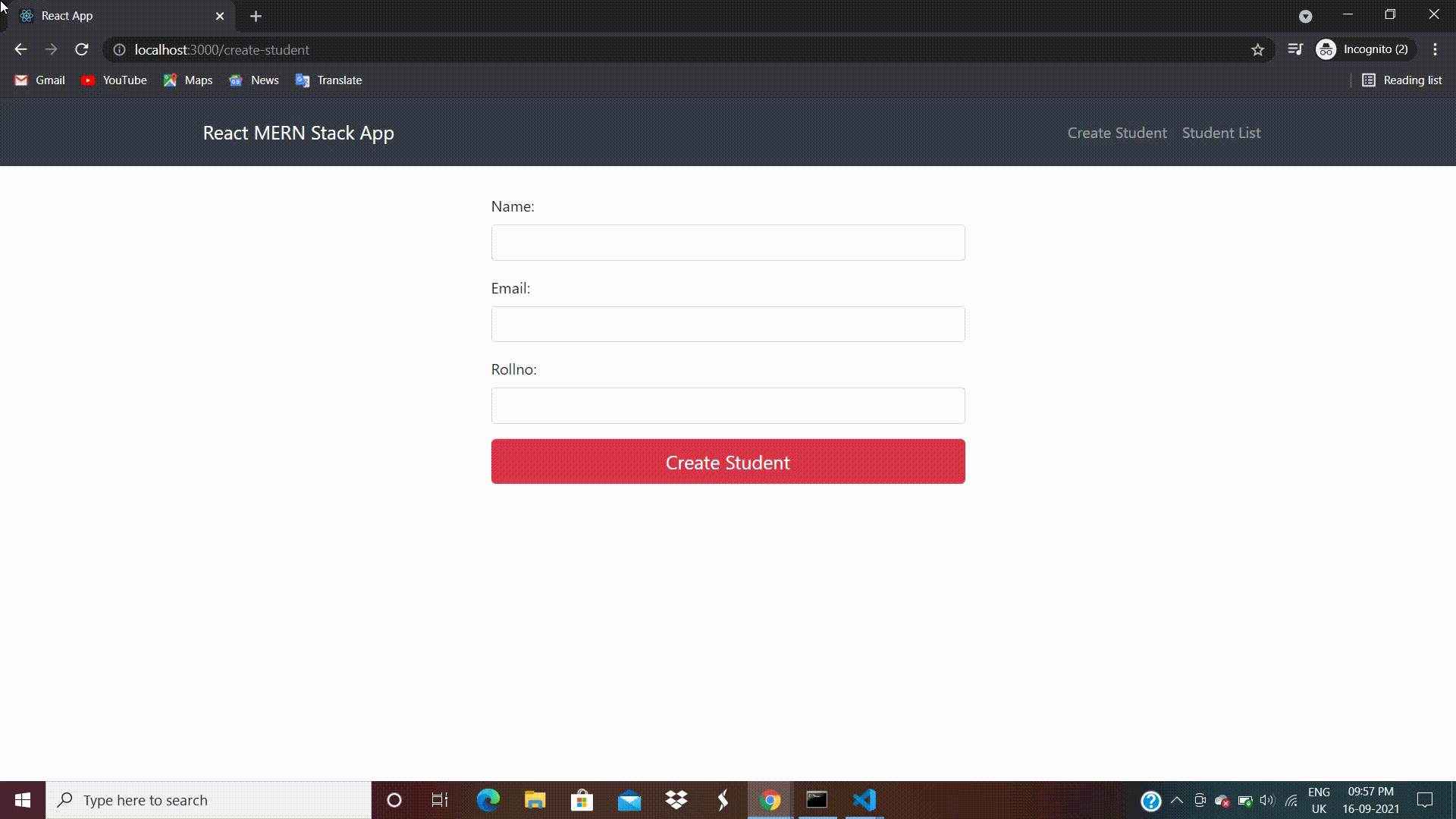Now we will work on the backend part of our application. We will create a folder inside our mern-stack-crud  to manage the server services such as database, models, schema, routes and APIs, name this folder backend.

Step 1: Run command to create backend folder for server and get inside of it.

`mkdir backend && cd backend`

Step 2: Create package.json – Next, we need to create a separate package.json file for managing the server of our mern-stack-crud  app.

`npm init -y`

Go to backend/package.json file will look like the following. Replace the test property like:

```"test": "echo \"Error: no test specified\" && exit 1"
"start": "nodemon server.js"```

Step 3: Install Node Dependencies – Install the following Node dependencies.

To install the above dependencies, run the following code on the terminal.

`npm install express body-parser cors mongoose`

You may install nodemon as dev dependency to automate the server restarting process.

`npm i -D nodemon`

Back-end project structure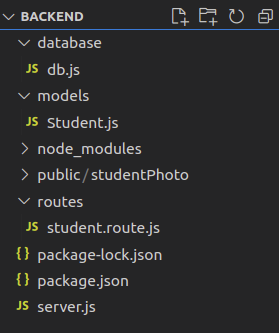back-end project structure

Step 4: Setting up MongoDB Database – In this step, we will set up a MongoDB database for our app. Before, starting make sure you have latest version of MongoDB is installed on your system. Create folder inside the backend  folder and name it database. Create a file by the name of db.js inside the database folder. Go to  backend/database/db.js and write the following code.

## db.js

 `module.exports = { ` `  ``db: ``'mongodb://localhost:27017/reactdb'` `};`

We have declared the MongoDB database and name it reactdb

Step 5: Define Mongoose Schema – Now, create MongoDB schema for interacting with MongoDB database. Create a folder called models inside backend folder to keep schema related files and create a file Student.js inside of it to define MongoDB schema. Go to  backend/models/Student.js and write the following code.

## Student.js

 `const mongoose = require(``'mongoose'``); ` `const Schema = mongoose.Schema; ` ` `  `let studentSchema = ``new` `Schema({ ` `  ``name: { ` `    ``type: String ` `  ``}, ` `  ``email: { ` `    ``type: String ` `  ``}, ` `  ``rollno: { ` `    ``type: Number ` `  ``} ` `}, { ` `    ``collection: ``'students'` `  ``}) ` ` `  `module.exports = mongoose.model(``'Student'``, studentSchema)`

We declared name, email, and rollno fields along with their respective data types in student Schema.

Step 6: Create Routes Using ExpressJS – In this step, we are set up some routes (REST APIs) for CREATE, READ, UPDATE and DELETE using Express and Node.js. These routes will help us to manage the data in our mern-stack-crud app. Create a folder and name it routes inside backend folder. Here we will keep all the routes related files. Also, create a file and name it student.routes.js inside routes folder, in this file we will define our routes.

`mkdir routes && cd routes && touch student.route.js`

Then, go to backend/routes/student.route.js file and write the following code.

## student.route.js

 `let mongoose = require(``"mongoose"``), ` `  ``express = require(``"express"``), ` `  ``router = express.Router(); ` ` `  `// Student Model ` `let studentSchema = require(``"../models/Student"``); ` ` `  `// CREATE Student ` `router.post(``"/create-student"``, (req, res, next) => { ` `  ``studentSchema.create(req.body, (error, data) => { ` `    ``if` `(error) { ` `      ``return` `next(error); ` `    ``} ``else` `{ ` `      ``console.log(data); ` `      ``res.json(data); ` `    ``} ` `  ``}); ` `}); ` ` `  `// READ Students ` `router.get(``"/"``, (req, res) => { ` `  ``studentSchema.find((error, data) => { ` `    ``if` `(error) { ` `      ``return` `next(error); ` `    ``} ``else` `{ ` `      ``res.json(data); ` `    ``} ` `  ``}); ` `}); ` ` `  `// UPDATE student ` `router ` `  ``.route(``"/update-student/:id"``) ` `  ``// Get Single Student ` `  ``.get((req, res) => { ` `    ``studentSchema.findById( ` `        ``req.params.id, (error, data) => { ` `      ``if` `(error) { ` `        ``return` `next(error); ` `      ``} ``else` `{ ` `        ``res.json(data); ` `      ``} ` `    ``}); ` `  ``}) ` ` `  `  ``// Update Student Data ` `  ``.put((req, res, next) => { ` `    ``studentSchema.findByIdAndUpdate( ` `      ``req.params.id, ` `      ``{ ` `        ``\$set: req.body, ` `      ``}, ` `      ``(error, data) => { ` `        ``if` `(error) { ` `          ``return` `next(error); ` `          ``console.log(error); ` `        ``} ``else` `{ ` `          ``res.json(data); ` `          ``console.log(``"Student updated successfully !"``); ` `        ``} ` `      ``} ` `    ``); ` `  ``}); ` ` `  `// Delete Student ` `router.``delete``(``"/delete-student/:id"``,  ` `(req, res, next) => { ` `  ``studentSchema.findByIdAndRemove( ` `      ``req.params.id, (error, data) => { ` `    ``if` `(error) { ` `      ``return` `next(error); ` `    ``} ``else` `{ ` `      ``res.status(200).json({ ` `        ``msg: data, ` `      ``}); ` `    ``} ` `  ``}); ` `}); ` ` `  `module.exports = router;`

Step 7: Configure server.js – We have almost created everything for our mern-stack-crud app. Now, create the server.js file in the root of the backend folder. Go to backend/server.js and write the following code.

## server.js

 `let express = require(``'express'``); ` `let mongoose = require(``'mongoose'``); ` `let cors = require(``'cors'``); ` `let bodyParser = require(``'body-parser'``); ` `let dbConfig = require(``'./database/db'``); ` ` `  `// Express Route ` `const studentRoute = require(``'../backend/routes/student.route'``) ` ` `  `// Configure mongoDB Database ` `mongoose.set(``'useNewUrlParser'``, ``true``); ` `mongoose.set(``'useFindAndModify'``, ``false``); ` `mongoose.set(``'useCreateIndex'``, ``true``); ` `mongoose.set(``'useUnifiedTopology'``, ``true``); ` ` `  `// Connecting MongoDB Database ` `mongoose.Promise = global.Promise; ` `mongoose.connect(dbConfig.db).then(() => { ` `  ``console.log(``'Database successfully connected!'``) ` `}, ` `  ``error => { ` `    ``console.log(``'Could not connect to database : '` `+ error) ` `  ``} ` `) ` ` `  `const app = express(); ` `app.use(bodyParser.json()); ` `app.use(bodyParser.urlencoded({ ` `  ``extended: ``true` `})); ` `app.use(cors()); ` `app.use(``'/students'``, studentRoute) ` ` `  ` `  `// PORT ` `const port = process.env.PORT || 4000; ` `const server = app.listen(port, () => { ` `  ``console.log(``'Connected to port '` `+ port) ` `}) ` ` `  `// 404 Error ` `app.use((req, res, next) => { ` `  ``res.status(404).send(``'Error 404!'``) ` `}); ` ` `  `app.use(``function` `(err, req, res, next) { ` `  ``console.error(err.message); ` `  ``if` `(!err.statusCode) err.statusCode = 500; ` `  ``res.status(err.statusCode).send(err.message); ` `});`

Now, we have successfully created the backend for our mern-stack-app.

Our final project directory structure: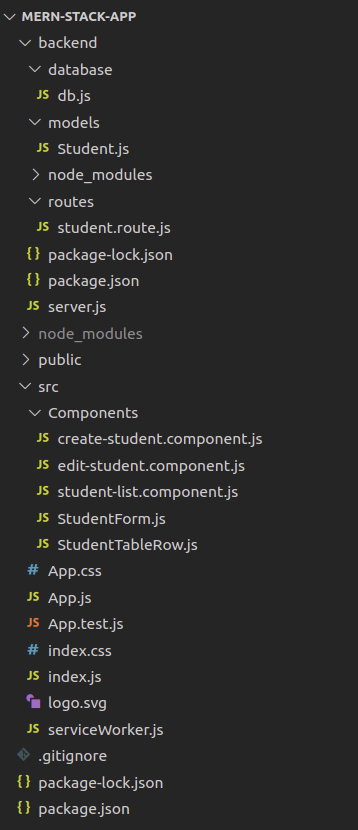project-directory-structure

Now, start the MongoDB database server to run the server.

Step to run the application: Open a terminal and run the following command to start the Nodemon server by staying in the backend folder.

`npm start`

If everything is working well you will see the following output on the terminal screen.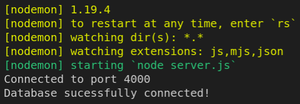mern-stack-crud server-running

Final output: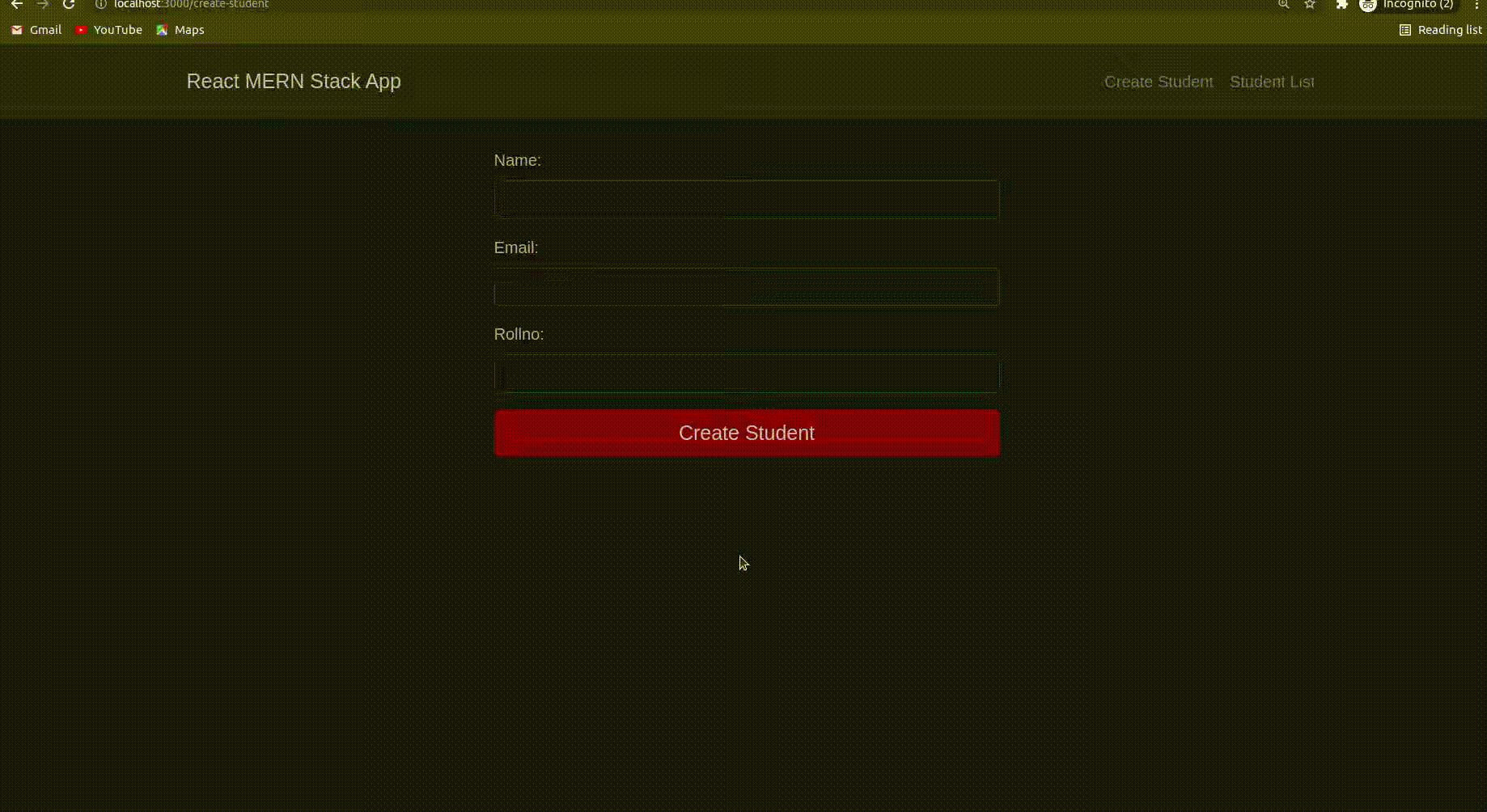mern-stack-crud-app

My Personal Notes arrow_drop_up
Related Articles